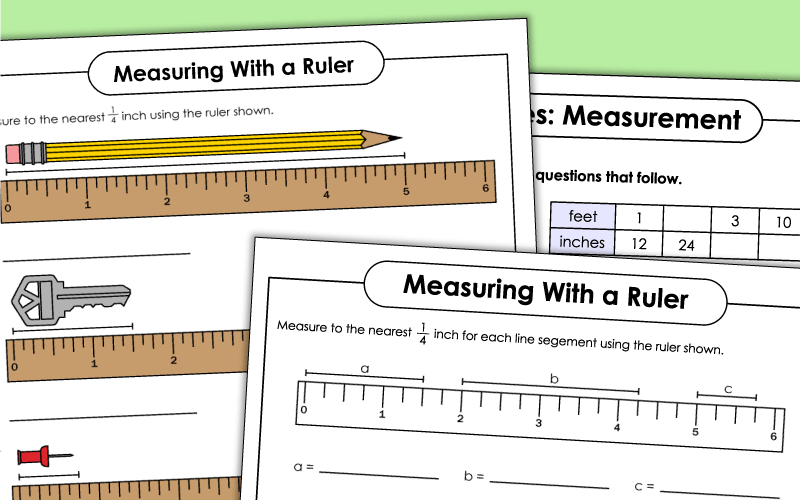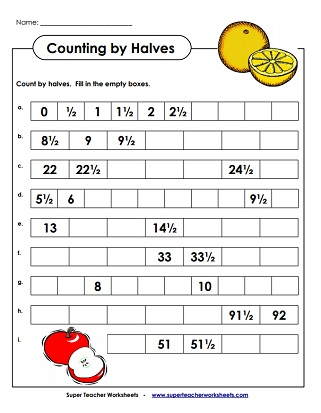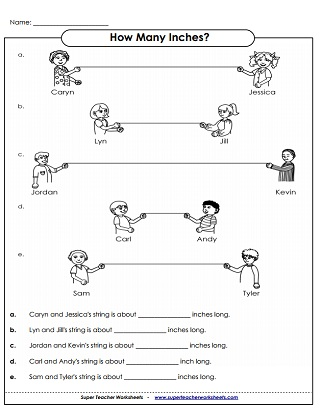# Measurement Worksheets: Yards, Feet, and Inches

Printable worksheets for standard American linear measurement, including inches, feet, and yards. Includes using rulers to measure to the nearest inch, half inch, quarter inch, and eighth inch. Also, there are worksheets for converting between feet, yards, and inches.## Nearest Inch

Measure Each String Picture to the Nearest Inch.
Draw a line that is 7 inches long. Draw another line that is 3 inches long. Subtract to find out how much longer the first line is than the second.

## Nearest Half Inch

Measure each shape using the ruler shown; Measure to the nearest half inch.
Measure each picture to the nearest half inch. using the given ruler.
Draw a line that is 7 inches long. Trace 4½ inches of the line with a yellow highlighter. Trace the rest with a blue highlighter. Find the length of the blue section.
Practice counting by halves.  Helps students learn to read rulers to the nearest half inch.

## Nearest Quarter Inch

Measure each object  using the ruler shown; Measure to the nearest quarter inch.
Measure each item  using the ruler shown to the nearest quarter inch.
State the length of each line segment to the nearest quarter inch.
Draw a line that is 7½ inches long. With a yellow marker trace 2¼ inches of the line. Trace the rest of the line with a green marker. Find the length of the green segment.
Practice counting by quarters.  Helps students learn to read rulers to the nearest quarter inch.

## Nearest Eighth Inch

Measure each item  using the graphic ruler; Measure to the nearest eighth inch.
State the length of each line segment to the nearest eighth inch.
Here's a picture of a ruler with fractional measurements labeled.
Practice counting by eighths. Fill in the missing numbers for each sequence.

## Conversions & Unit Comparisons

Complete the table and answer the standard measurement word problems.
This worksheet has 12 simple conversion problems and 2 word problems.
Help students work on their conversion skills with this worksheet, which includes fractions of halves and thirds.
These basic conversions are made up entirely of whole numbers.
Have students practice converting between inches, feet, yards, and miles with this worksheet. It includes 10 simple conversion problems and 2 word problems.
This worksheet has several styles of questions, including a table to fill in, word problems, and simple conversions.
This variety worksheet includes larger numbers than the basic worksheet, fractions, and multi-step word problems.
Use these task cards to help students practice a variety of problems on converting inches, feet, and yards.
Set of four measurement bookmarks for reference.  Includes linear measurement (standard and metric) and capacity.
Use the "greater than" and "less than" symbols to compare measurements in feet and inches.
Determine which measurement is not equivalent to the other two.
A take-home project that requires students to accurately measure friends or family members.
In-and-out boxes for converting yards, feet, and inches; includes word problems.
Cut out the flower flash cards. Measure each flower.
Measurement learning center that's easy to set up.

## Word Problems

These word problems reinforce math skills having to do with converting inches, feet, and yards.
These word problems include a mix of inches, feet, and yards.

Metric Measurement (Centimeters, Millimeters)

Metric measurement with a ruler; Measure objects to the nearest centimeter or millimeter.

Capacity Worksheets

Measuring volume with gallons, quarts, pints, and cup.

In-Out Boxes

Worksheets with in-out boxes at different levels.

## Sample Worksheet ImagesMy Account
Site Information